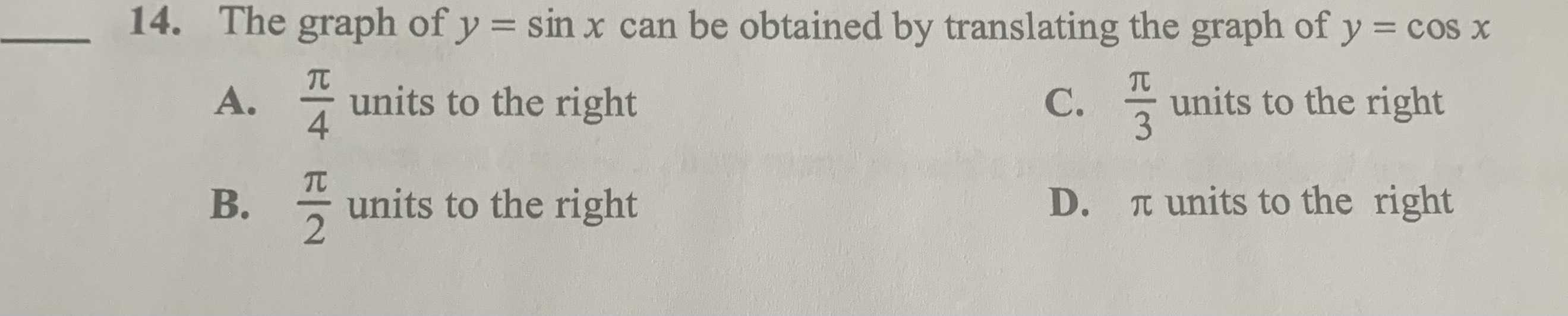### Still have math questions?

Trigonometry
QuestionThe graph of $$y = \sin x$$ can be obtained by translating the graph of $$y = \cos x$$

A. $$\frac { \pi } { 4 }$$ units to the right C. $$\frac { \pi } { 3 }$$ units to the right B. $$\frac { \pi } { 2 }$$ units to the right D. $$\pi$$ units to the right